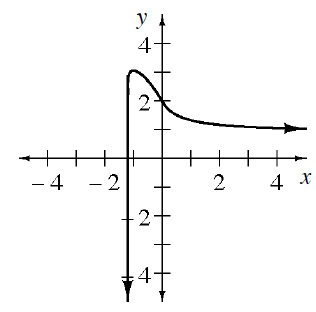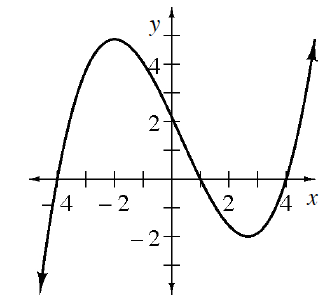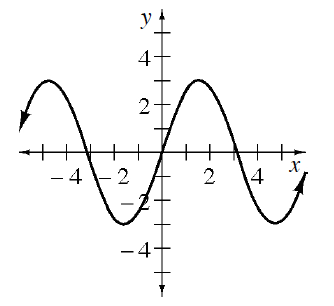### Home > APCALC > Chapter 6 > Lesson 6.4.1 > Problem6-125

6-125.

For each graph below:

1. Trace the curve on your paper and write a slope statement.

2. Sketch the graph of the derivative using a different color.

1.1.1.Slope statements should tell a story, starting at the left side of the graph and ending at the right side.
There should be a description of where the function is increasing or decreasing and how the rate in which it is increasing or decreasing is changing.

Steep positive slopes on the graph of $y = f(x)$ should have very large $y$-values on the graph of $y = f ^\prime(x)$. Steep negative slopes on the graph of $y = f(x)$ should have very large negative $y$-values on the graph of $y = f ^\prime(x)$. Maxima and minima on $y = f(x)$ have tangent lines with slopes of $0$, so they are roots on the graph of $y = f(x)$.